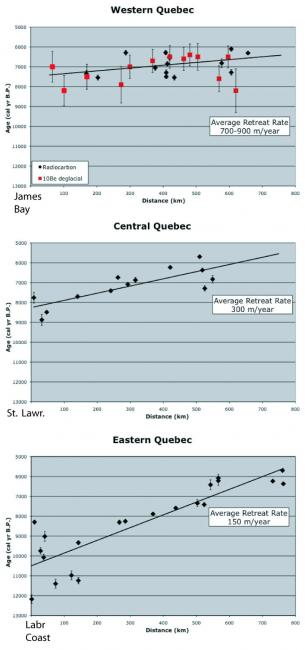Figure 3. Retreat rate plots.View Original Image at Full Size

Figure 3. Age vs. Distance plots for each data transect: Western (top), Central (middle), and Eastern Quebec (bottom). Black points are from radiocarbon dates (Dyke, 2004). Red points in the top plot are from surface exposure dates (Carlson et al., 2007). Retreat rates are calculated using the slope of linear fit in the data and are listed in the white box in each plot.

Image 31017 is a 1523 by 715 pixel JPEG